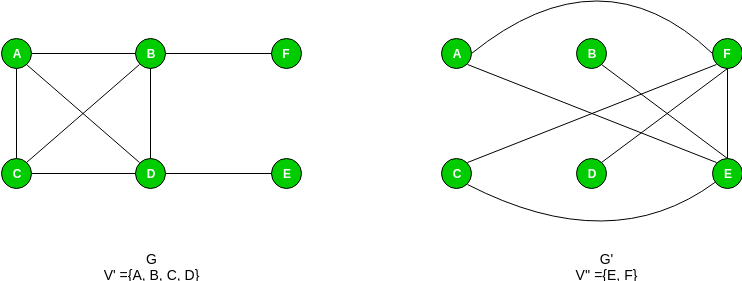# Proof that vertex cover is NP complete

Prerequisite – Vertex Cover Problem, NP-Completeness
Problem – Given a graph G(V, E) and a positive integer k, the problem is to find whether there is a subset V’ of vertices of size at most k, such that every edge in the graph is connected to some vertex in V’.

Explanation –
First let us understand the notion of an instance of a problem. An instance of a problem is nothing but an input to the given problem. An instance of the Vertex Cover problem is a graph G (V, E) and a positive integer k, and the problem is to check whether a vertex cover of size at most k exists in G. Since an NP Complete problem, by definition, is a problem which is both in NP and NP hard, the proof for the statement that a problem is NP Complete consists of two parts:

1. Proof that vertex cover is in NP –
If any problem is in NP, then, given a ‘certificate’ (a solution) to the problem and an instance of the problem (a graph G and a positive integer k, in this case), we will be able to verify (check whether the solution given is correct or not) the certificate in polynomial time.

The certificate for the vertex cover problem is a subset V’ of V, which contains the vertices in the vertex cover. We can check whether the set V’ is a vertex cover of size k using the following strategy (for a graph G(V, E)):

```let count be an integer
set count to 0
for each vertex v in V’
remove all edges adjacent to v from set E
increment count by 1
if count = k and E is empty
then
the given solution is correct
else
the given solution is wrong
```

It is plain to see that this can be done in polynomial time. Thus the vertex cover problem is in the class NP.

2. Proof that vertex cover is NP Hard –
To prove that Vertex Cover is NP Hard, we take some problem which has already been proven to be NP Hard, and show that this problem can be reduced to the Vertex Cover problem. For this, we consider the Clique problem, which is NP Complete (and hence NP Hard).

“In computer science, the clique problem is the computational problem of finding cliques (subsets of vertices, all adjacent to each other, also called complete subgraphs) in a graph.”

Here, we consider the problem of finding out whether there is a clique of size k in the given graph. Therefore, an instance of the clique problem is a graph G (V, E) and a non-negative integer k, and we need to check for the existence of a clique of
size k in G.

Now, we need to show that any instance (G, k) of the Clique problem can be reduced to an instance of the vertex cover problem. Consider the graph G’ which consists of all edges not in G, but in the complete graph using all vertices in G. Let us call this the complement of G. Now, the problem of finding whether a clique of size k exists in the graph G is the same as the problem of finding whether there is a vertex cover of size |V| – k in G’. We need to show that this is indeed the case.

Assume that there is a clique of size k in G. Let the set of vertices in the clique be V’. This means |V’| = k. In the complement graph G’, let us pick any edge (u, v). Then at least one of u or v must be in the set V – V’. This is because, if both u and v were from the set V’, then the edge (u, v) would belong to V’, which, in turn would mean that the edge (u, v) is in G. This is not possible since (u, v) is not in G. Thus, all edges in G’ are covered by vertices in the set V – V’.

Now assume that there is a vertex cover V’’ of size |V| – k in G’. This means that all edges in G’ are connected to some vertex in V’’. As a result, if we pick any edge (u, v) from G’, both of them cannot be outside the set V’’. This means, all
edges (u, v) such that both u and v are outside the set V’’ are in G, i.e., these edges constitute a clique of size k.

Thus, we can say that there is a clique of size k in graph G if and only if there is a vertex cover of size |V| – k in G’, and hence, any instance of the clique problem can be reduced to an instance of the vertex cover problem. Thus, vertex cover is NP Hard. Since vertex cover is in both NP and NP Hard classes, it is NP Complete.

To understand the proof, consider the following example graph and its complement:Attention reader! Don’t stop learning now. Get hold of all the important DSA concepts with the DSA Self Paced Course at a student-friendly price and become industry ready.

My Personal Notes arrow_drop_upCheck out this Author's contributed articles.

If you like GeeksforGeeks and would like to contribute, you can also write an article using contribute.geeksforgeeks.org or mail your article to contribute@geeksforgeeks.org. See your article appearing on the GeeksforGeeks main page and help other Geeks.

Please Improve this article if you find anything incorrect by clicking on the "Improve Article" button below.

5

Please write to us at contribute@geeksforgeeks.org to report any issue with the above content.